# Go Math Grade 4 Answer Key Homework FL Chapter 13 Algebra: Perimeter and Area Review/Test

Go Math Grade 4 Answer Key Homework FL Chapter 13 Algebra: Perimeter and Area Review/Test helps the students enhance their math skills and gain knowledge on the perimeter and area of the different shapes. The solutions given in this chapter are prepared by the math experts. By following the Go Math Grade 4 Answer key, students can easily find out the Perimeter and Area of the rectangle, square, and etc.

## Go Math Grade 4 Answer Key Homework FL Chapter 13 Algebra: Perimeter and Area Review/Test

Go Math Answer Key will give you the perfect answers with a clear explanation of every question in an easy way. Go Math explained clearly about Perimeter and Area of different Shapes by using images, indicating images with arrows, and numbers.

Chapter: 13 – Review/Test

### Review/Test – Page No. 519

Choose the best term from the box.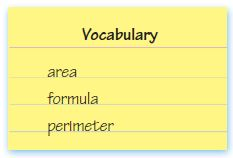Question 1.
The number of square units needed to cover a flat surface is the ______________.
_________

The number of square units needed to cover a flat surface is the area.

Question 2.
The distance around a shape is the ____________.
_________

The distance around a shape is the Perimeter.

Find the area of the rectangle or combined rectangles.

Question 3.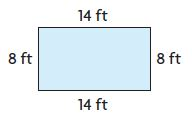A = _____ square feet

Explanation:
Given,
length = 14 ft
Width = 8 ft
Area of the rectangle = l × w
A = 14 ft × 8 ft
A = 112 square feet
Thus the area of the rectangle for the above figure is 112 square feet.

Question 4.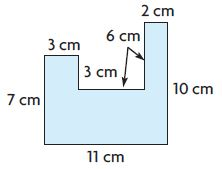A = _____ square centimeters

Explanation:
Figure 1:
S = 3 cm
Area of the square = s × s
A = 3 cm × 3 cm
A = 9 square cm
Figure 2:
b = 11 cm
h = 4 cm
Area of the rectangle = b × h
A = 11 cm × 4 cm
A = 44 square cm
Figure 3:
b = 2 cm
h = 6 cm
Area of the rectangle = b × h
A = 2 cm × 6 cm
A = 12 square cm
Area of the composite figure is 9 sq. cm + 44 sq. cm + 12 sq. cm = 65 square cm.

Find the unknown measure of the rectangle.

Question 5.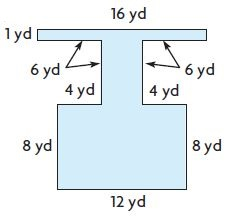A = _____ square yards

Explanation:
Figure 1:
b = 12 yd
h = 8 yd
Area of the rectangle = b × h
A = 12 yd × 8 yd
A = 96 square yard.
Figure 2:
b = 16 yd
h = 1 yd
Area of the rectangle = b × h
A = 16 yd × 1 yd
A = 16 square yard.
Figure 3:
b = 4 yd
h = 6 yd
Area of the rectangle = b × h
A = 4 yd × 6 yd
A = 24 square yard.
The area of the composite figure is 96 square yard + 16 square yard + 24 square yard = 136 square yard.

Question 6.Perimeter = 60 meters
width = _____ m

Explanation:
Given,
Perimeter = 60 meters
length = 18 m
width = _____ m
The perimeter of the rectangle = l + w + l + w
P = 2l + 2w
60 m = 2 × 18 m + 2w
60 m – 36 m = 2w
2w = 24
w = 24/2
w = 12 meters
Thus the width of the above rectangle is 12 meters.

Question 7.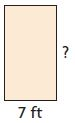Area = 91 square feet
height = _____ feet

Explanation:
Given,
Area = 91 square feet
base = 7 ft
height = _____ feet
Area of the rectangle = b × h
91 sq ft = 7 ft × h
h = 91/7
h = 13 ft
Thus the height of the above rectangle is 13 ft.

Question 8.Area = 60 square inches
base = _____ in.

Explanation:
Given,
Area = 60 square inches
height = 6 in
base = _____ in.
Area of the rectangle = b × h
60 square inches = b × 6 in
b = 60/6
b = 10 inches
Thus the base of the above rectangle is 10 inches.

Question 9.
What is the perimeter of a rectangle with a length of 13 feet and a width of 9 feet?
P = _____ ft

Explanation:
Given,
l = 13 ft
w = 9 ft
The perimeter of the rectangle = l + w + l + w
P = 13 ft + 9 ft + 13 ft + 9 ft
P = 44 ft
Thus the perimeter of the rectangle is 44 ft.

### Review/Test – Page No. 520

Question 10.
Which pair of shapes has the same area?
Options:
a.b.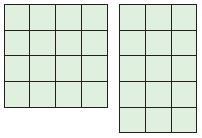c.d.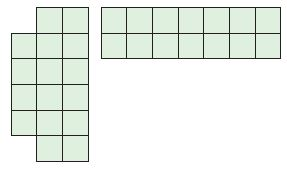Answer:Explanation:
a.4 × 2 = 8
3 × 3 = 9
8 ≠ 9
b.4 × 4 = 16
3 × 5 = 15
16 ≠ 15
c.3 × 4 = 12
2 × 6 = 12
12 = 12
Thus the correct answer is option c.

Question 11.
Jamie’s mom wants to enlarge her rectangular garden by adding a new rectangular section. The garden is now 96 square yards. What will the total area of the garden be after she adds the new section?
Options:
a. 84 square yards
b. 96 square yards
c. 180 square yards
d. 192 square yards

Explanation:
Given that,
Jamie’s mom wants to enlarge her rectangular garden by adding a new rectangular section. The garden is now 96 square yards.
Add 96 square yards to the rectangular garden.
96 square yards + 96 square yards = 192 square yards
Thus the correct answer is option d.

Question 12.
A rectangular yoga studio has an area of 153 square feet. The width of the studio is 9 feet. What is the length of the studio?
lenght = _____ ft

Explanation:
Given,
A rectangular yoga studio has an area of 153 square feet.
The width of the studio is 9 feet.
Area of the rectangle = l × w
153 square feet = l × 9 ft
l = 153/9
l = 17 ft
Therefore the length of the studio is 17 feet.

### Review/Test – Page No. 521

Question 13.
Mr. Patterson had a rectangular deck with an area of 112 square feet built in his backyard. Which could be a diagram of Mr. Patterson’s deck?
Options:
a.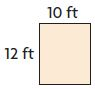b.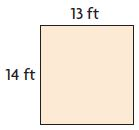c.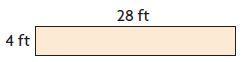d.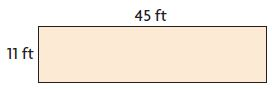Answer: c.Explanation:
Given,
Mr. Patterson had a rectangular deck with an area of 112 square feet built in his backyard.
Area of the rectangle = l × w
A = 28 ft × 4 ft
A = 112 square feet
Thus the correct answer is option c.

Question 14.
The town indoor pool is in a rectangular building. Marco is laying tile around the rectangular pool. How many square meters of tile will Marco need?Options:
a. 96 square meters
b. 252 square meters
c. 572 square meters
d. 892 square meters

Explanation:
The outer rectangle is
l = 26 m
w = 22 m
Area of the rectangle = l × w
A = 26 m × 22 m
A = 572 square meters
The inner rectangle is
l = 20 m
w = 16 m
Area of the rectangle = l × w
A = 20 m × 16 m
A = 320 square meters
Thus the square meters of tile will Marco need is 572 – 320 = 252 square meters.

### Review/Test – Page No. 522

Question 15.
A drawing of a high school pool is shown below.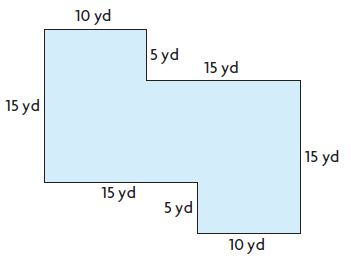What is the area of the pool? Explain how you know.
_______ square yards

Explanation:
Figure 1:
l = 10 yd
w = 15 yd
Area of the rectangle = l × w
A = 10 yd × 15 yd
A = 150 square yard
Figure 2:
l = 15 yd
w = 10 yd
Area of the rectangle = l × w
A = 15 yd × 10 yd
A = 150 square yard
Figure 3:
l = 10 yd
w = 5 yd
Area of the rectangle = l × w
A = 10 yd × 5 yd
A = 50 square yard
Area of the pool = 150 square yard + 150 square yard + 50 square yard = 350 square yard

Question 16.
Mr. Brown has 24 meters of fencing. He wants to build a rectangular pen for his rabbits.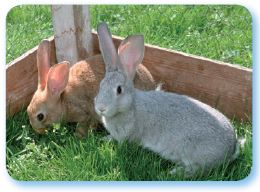A. Draw two different rectangles that Mr. Brown could build. Use only whole numbers for the lengths of the sides of each rectangle. Label the length of each side.
Type below:
_________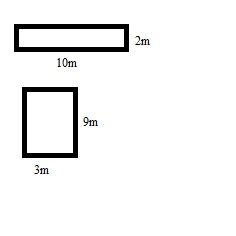Question 16.
B. Find the area in square meters of each rabbit pen you made in Part A. Show your work.
Type below:
_________

Explanation:
l = 10m
w = 2m
Area of the rectangle = l × w
A = 10 m × 2 m
A = 20 square meters
Therefore the area in square meters of each rabbit pen is 20 square meters.

Question 16.
C. If you were Mr. Brown, which of the two pens above would you construct for your rabbits? Explain why.
Type below:
_________

Answer: I would construct the second figure for the two rabbit pens.

Conclusion:
By following the Go Math Grade 4 Review Test solutions, students can quickly find the perimeter and area of shapes within a few minutes. Keep in touch with us to get the Go Math Grade 4 Answer Key Chapter 13 Algebra: Perimeter and Area.

Scroll to Top